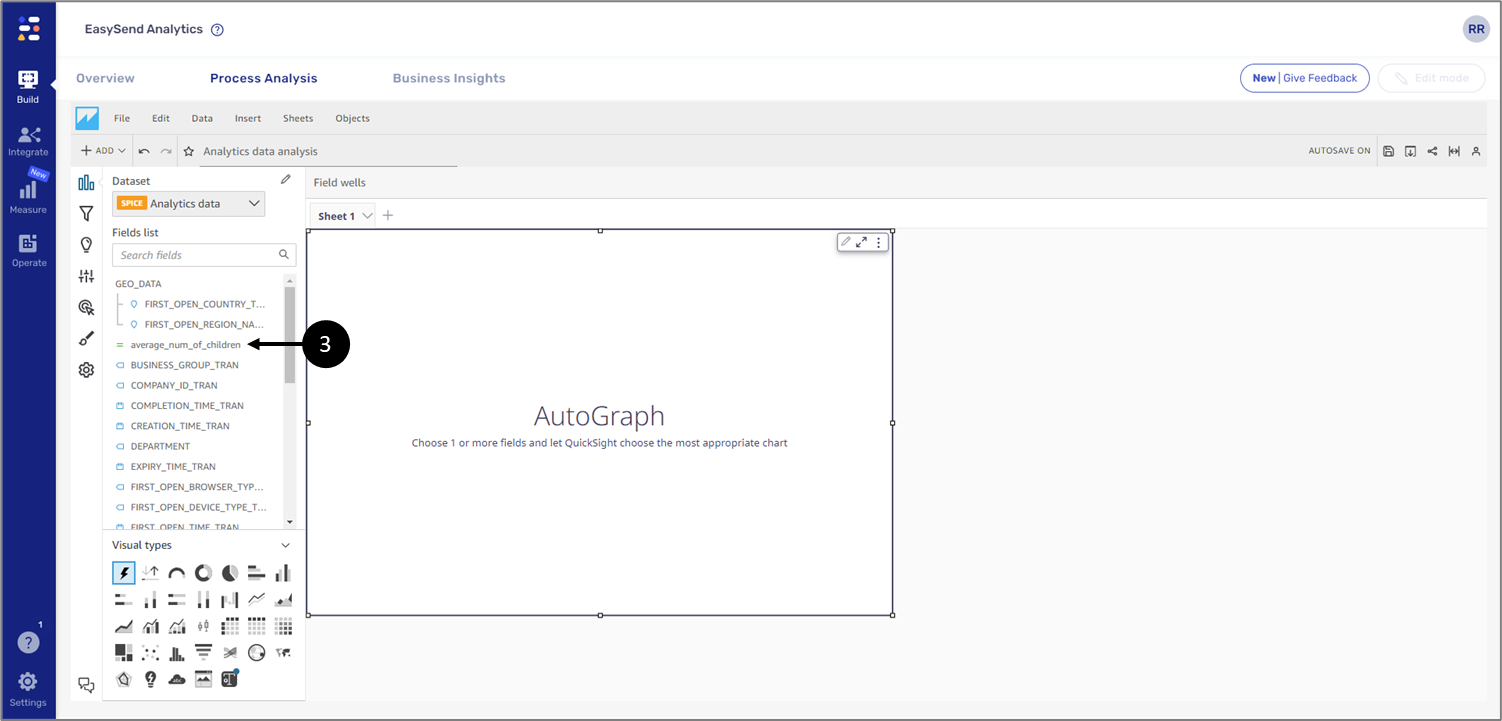Datasets and Fields
• PDF

# Datasets and Fields

• PDF

Article Summary

## Article Overview

The following article describes how to use datasets, visuals, and fields within an analysis. The article also describes how to add a calculated field.

## Datasets

(See Figure 1)

After creating an analysis as described in the Analysis Overview article, it appears with one selected dataset (1). A dataset can be replaced (2). The system displays the import status of the dataset fields (3). Additional datasets can be added by clicking the pencil icon (4). After clicking the icon, the “Dataset in this analysis window” appears.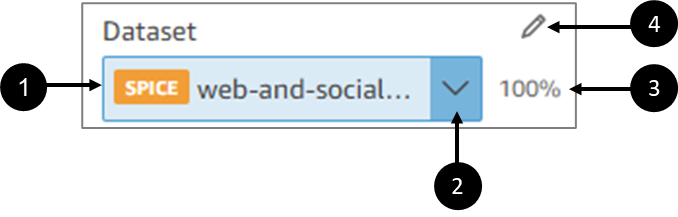### Datasets in this analysis Window

Figure 2 and Table 1 describe the structure of the Datasets in this analysis window.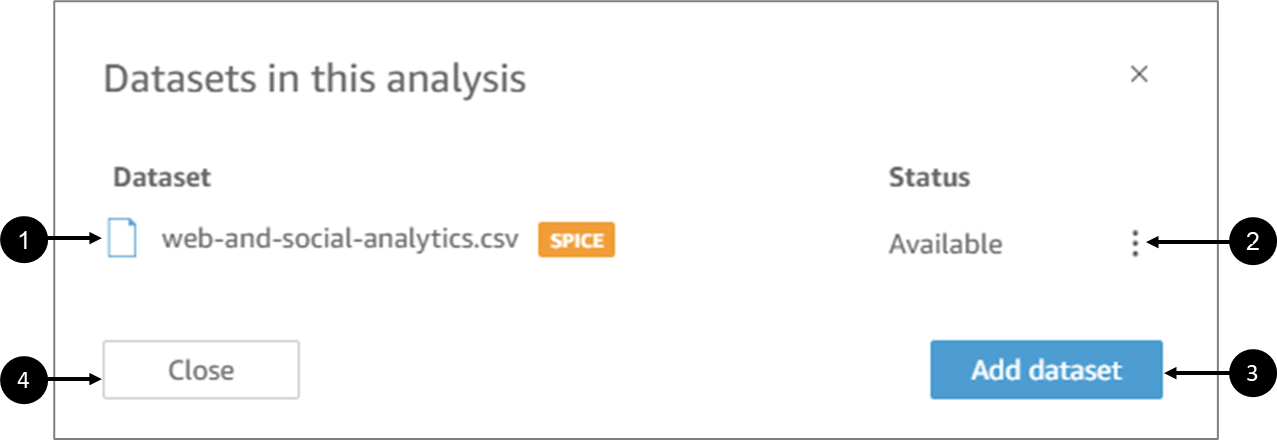Figure 2: Datasets in this analysis Window

Table 1: Datasets in this analysis Window

 Number Icon Name Description 1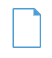Current dataset Displays the name and type of the current dataset 2 - Available Enables to replace the dataset 3 - Add dataset Enables to add additional datasets to the analytics 4 - Close Closes the Datasets in this analysis window

## Fields

(See Figure 3)

Each dataset contains fields with data that will be visualized. These are located under the Fields list (1).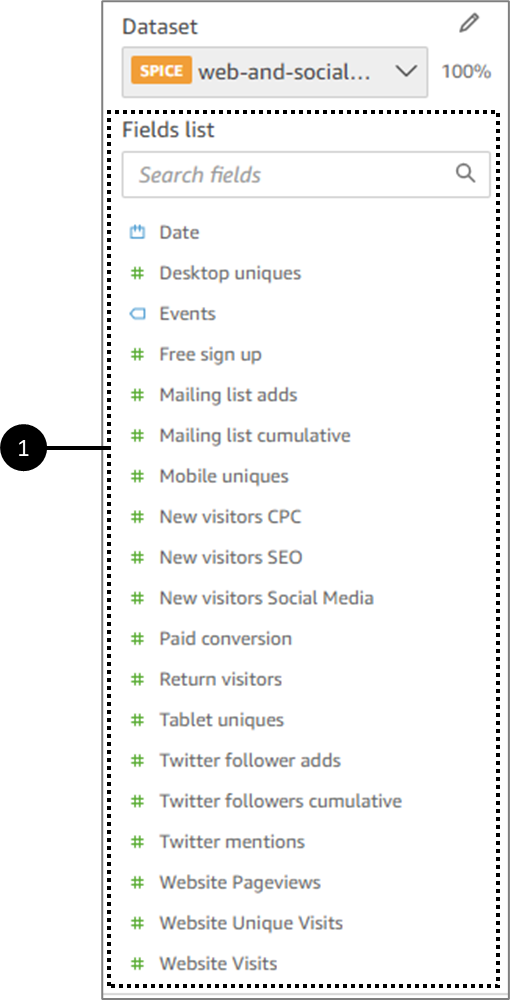Figure 3: Fields list

When an analysis contains more than one dataset when switching between them, the Fields list updates.

### Fields - Dimensions and Measures

(See Figure 4 and Figure 5)

Each field in a dataset is defined as a dimension or measure, depending on the type of data it contains.

In the Fields list, dimension fields have blue icons (1), and measure fields have green icons (2):

• Dimensions are text or date fields that can be items like products, or attributes that are related to measures. You can use dimensions to partition these items or attributes, like sales dates for sales figures.
• Measures are numeric values that are used for measurement, comparison, and aggregation.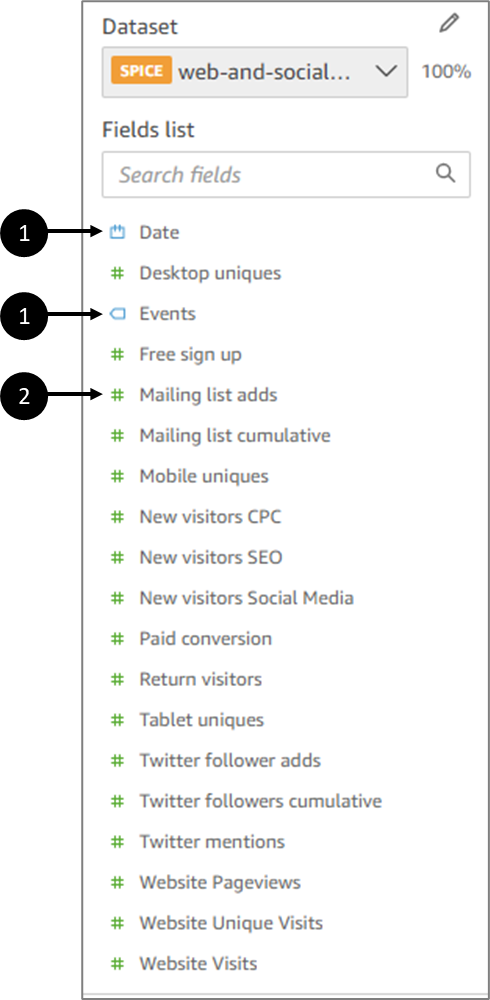Figure 4: Fields list

In some cases, EasySend Analytics interprets a field as a measure that you want to use as a dimension (or the other way around). If so, you can change the setting for that field.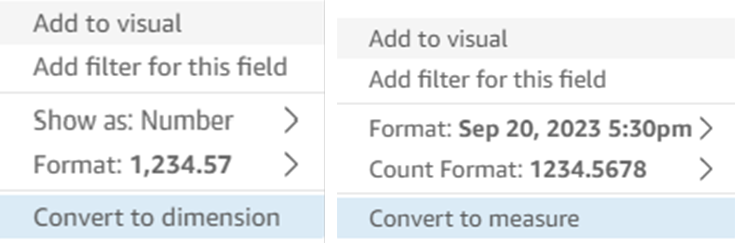Figure 5: Conversion Options

Changing a field's measure or dimension setting changes it for all visuals in the analysis that use that dataset. However, it doesn't change it in the dataset itself. You typically use a combination of dimension and measure fields to produce a visual, for example, sales totals (a measure) by sales date (a dimension).

## How to Create Visuals

(See Figure 6)

To visualize data in an analysis you must create a visual and then add fields.

You can create a visual in several ways:

• Select the fields that you want and use AutoGraph (1) to let EasySend Analytics determine the most appropriate visual type.
• Choose a specific visual from the Visual types (2) section.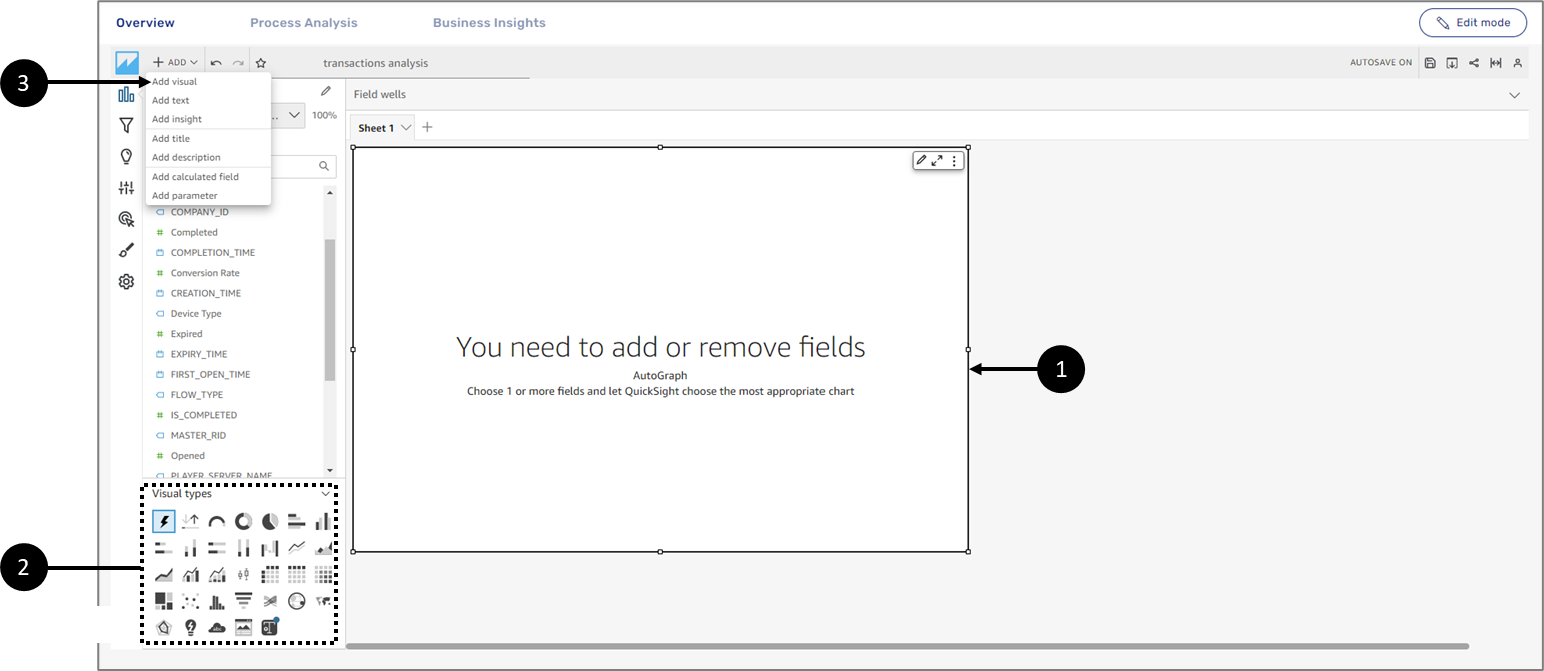Figure 6: Creating a Visual

To create a useful visual, it helps to know what question you are trying to answer as specifically as possible. It also helps to use the smallest dataset that can answer that question. Doing so helps you create simpler visuals that are easier to analyze.

## Adding Fields to a Visual

The following sections describe examples of adding fields to a visual.

### Adding a Field by Clicking it in the Fields list

When clicking a field from the Fields list, EasySend Analytics maps the field to the most appropriate visual element. EasySend Analytics adds the field to the visual by populating the first empty field well that corresponds with that field type (either measure or dimension). If all the visual elements are already populated, EasySend Analytics determines the most appropriate field well and replaces the field in it with the field you selected.

### Adding a Field by Using a Drop Target

(See Figure 7)

To add a field to a visual by using a drop target, first, choose a field in the Fields list pane. Then drag the field to your chosen drop target on the visual, making sure the drop indicator shows that the field is being added.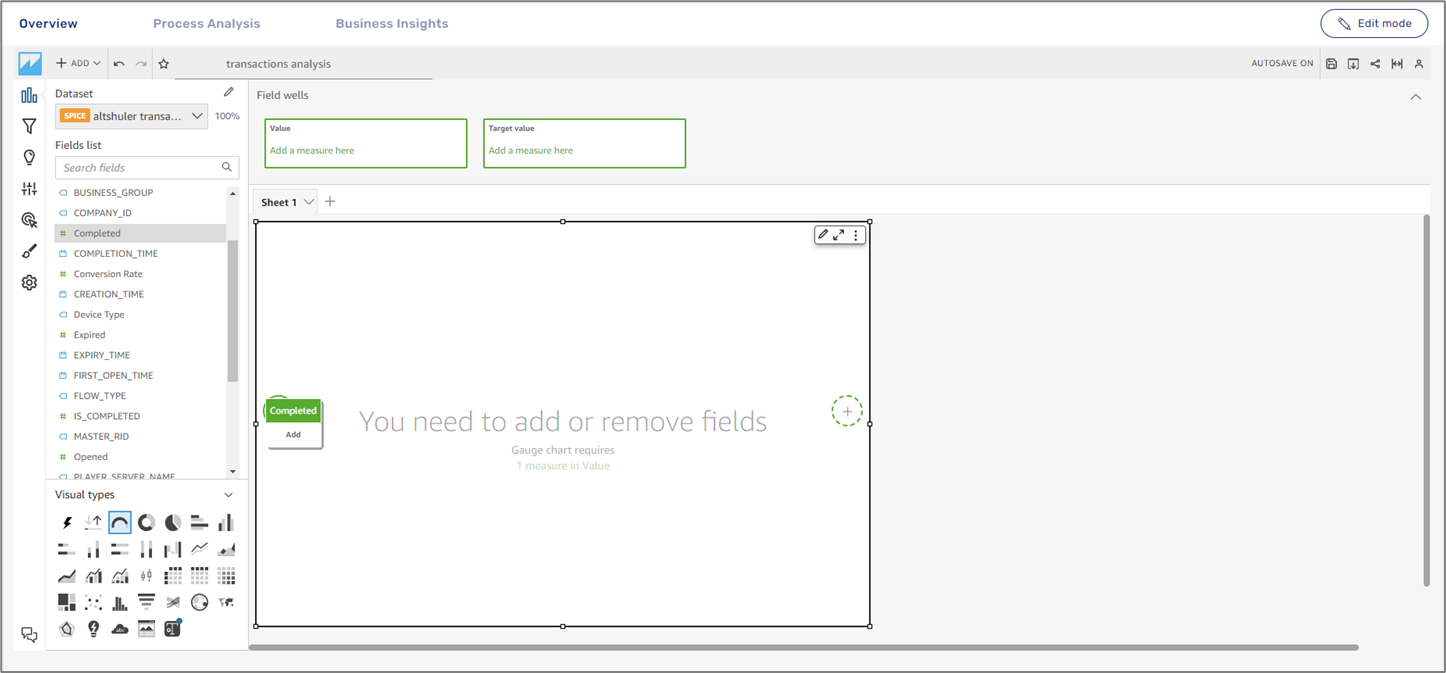Figure 7: Visual Drop Target

### Adding a Field by Using Field Wells

(See Figure 8)

To add a field to a visual by using Field wells, click to expand them. Choose a field in the Fields list pane. Drag the field to the target location while making sure that the drop indicator shows that the field is being added.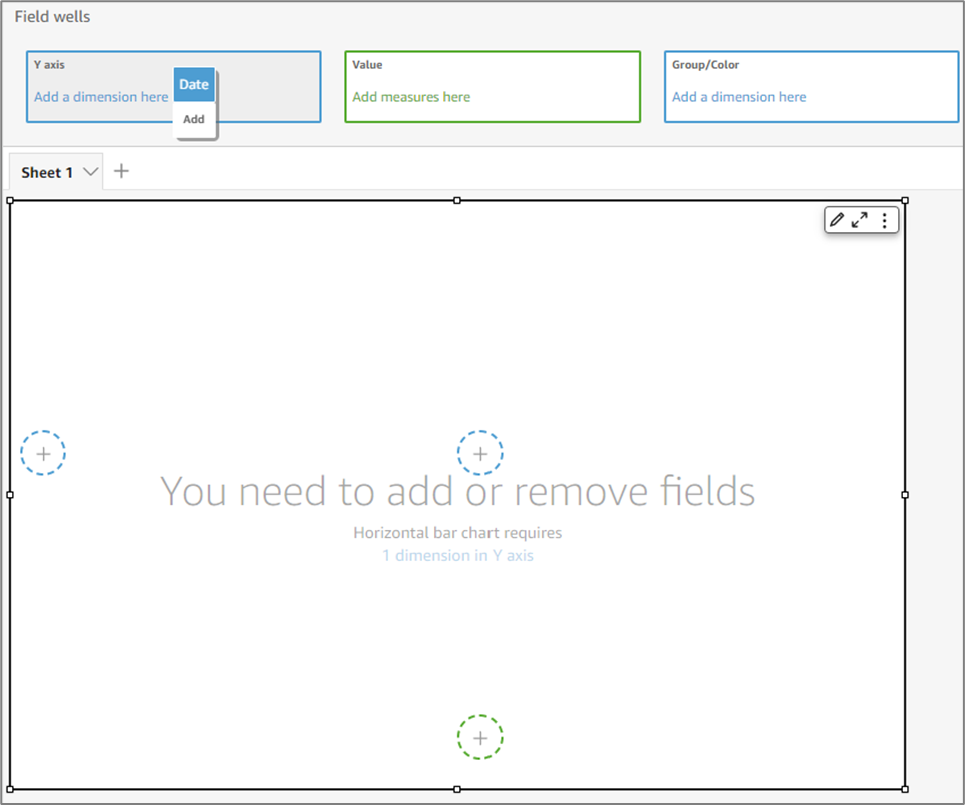Figure 8: Field wells

### Adding Fields to the AutoGraph Visual Example

If the visual you are adding fields to is an AutoGraph, click or drag and drop a field directly into the visual and let EasySend analytics choose the most appropriate visual. In the following example, the clicked field from the Fields list was Events (1), a dimension, and the visual that was automatically selected by the AutoGraph was a horizontal bar chart (2) (see Figure 9):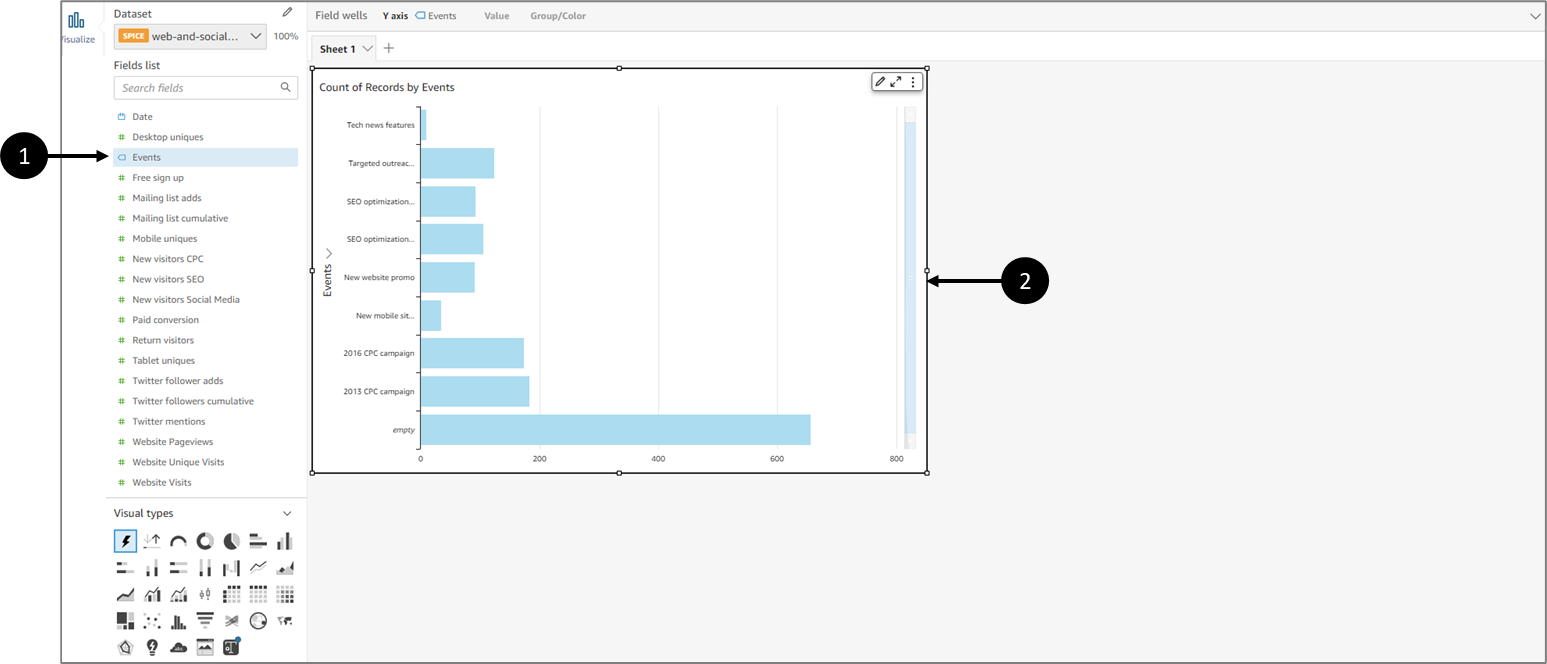Figure 9: AutoGraph Visual Example

### Adding Fields to a Visual (Not AutoGraph) Example

If the visual you are adding fields to is not an AutoGraph, first click to choose a visual from the Visual types section (1). Next, add fields from the Fields list by:

• Clicking
• Drag and drop into the visual
• Drag and drop into the Field wells

In the following example the selected visual was horizontal bar chart (1) and the selected field from the Fields list was Events (1) (see Figure 10):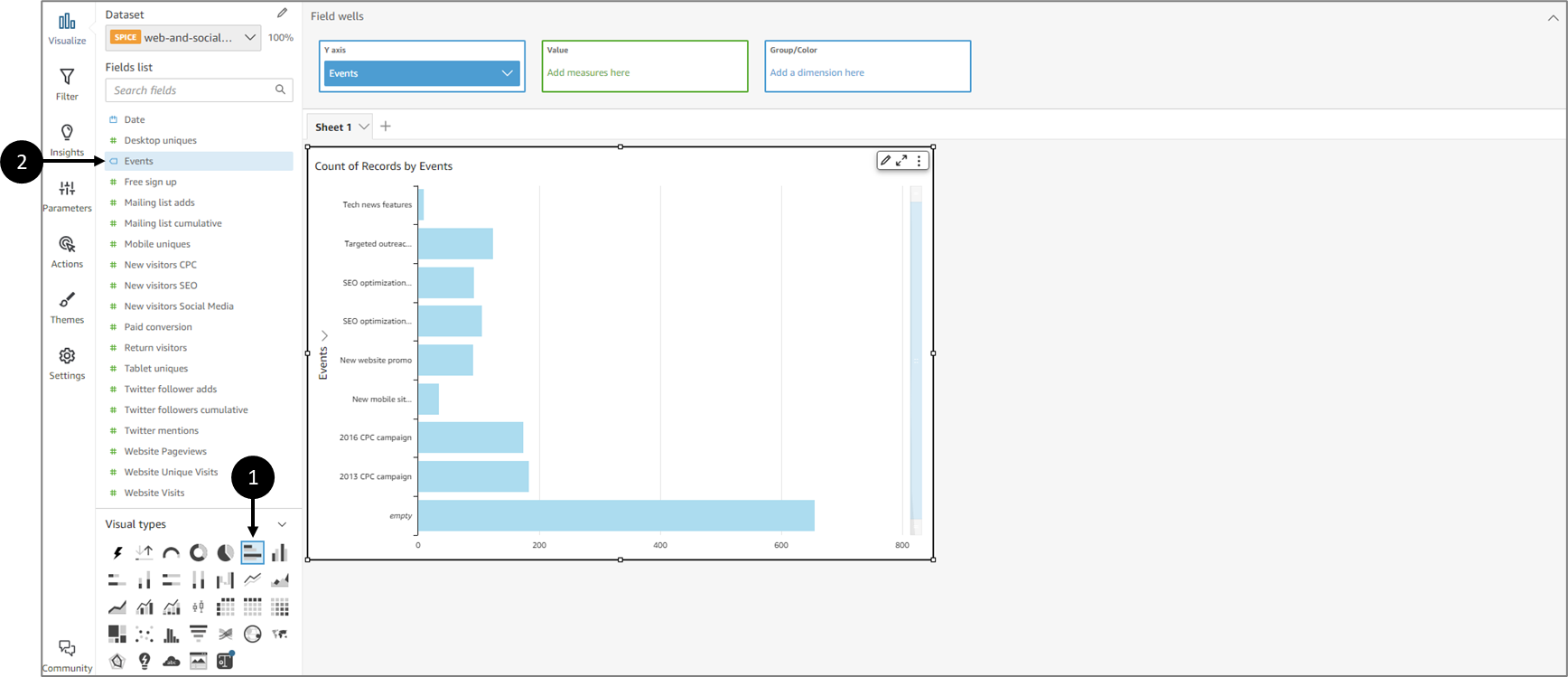Figure 10: Horizontal Bar chart Visual Example

## Field wells

(See Figure 11)

Field wells are different types of measures (green) and dimensions (blue) that appear according to the selected visual, for example:

• Measures:
• X-axis
• Y-axis
• Size
• Value
• Target value
• Dimensions:
• Group by
• Color
• Group/Color
• X-axis
• Trend group
• Breakdown
• Small multiples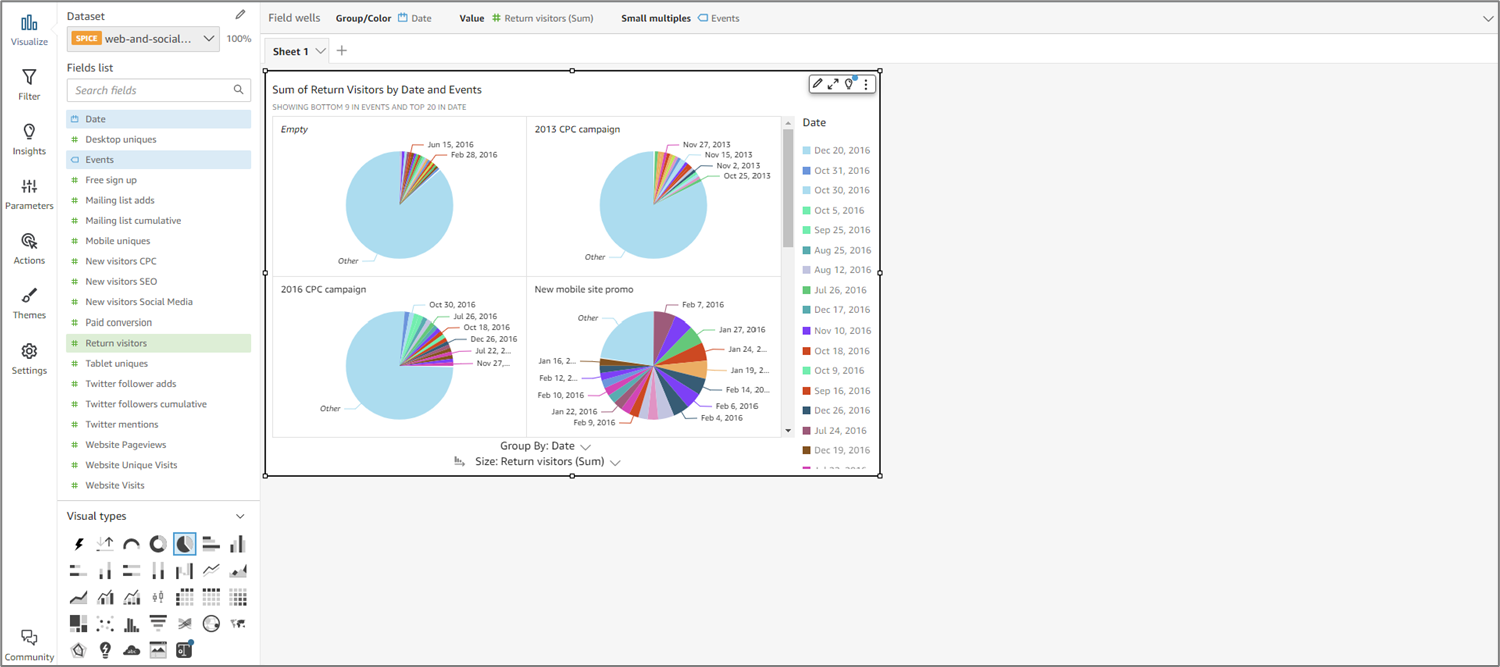Figure 11: Field wells

### Editing Field wells

(See Figure 12 and Figure 13)

To edit Field wells, it is mandatory to click and expand their section, for example: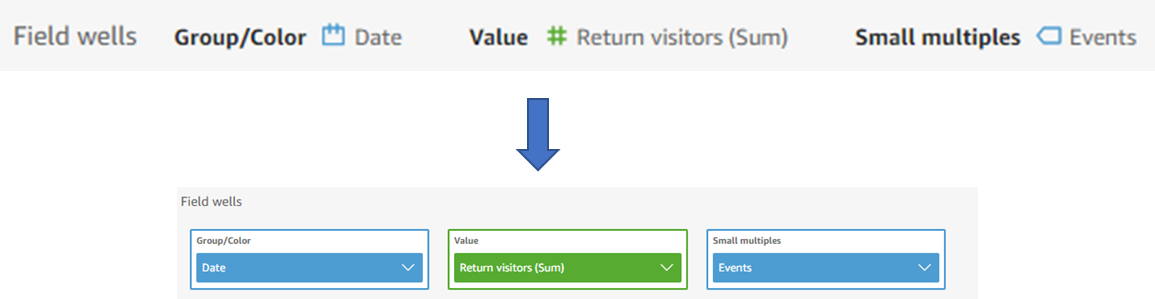Figure 12: Expanded Field wells

Each field well has a dropdown that displays different options, for example:

• Aggregating
• Sorting
• Display and formatting options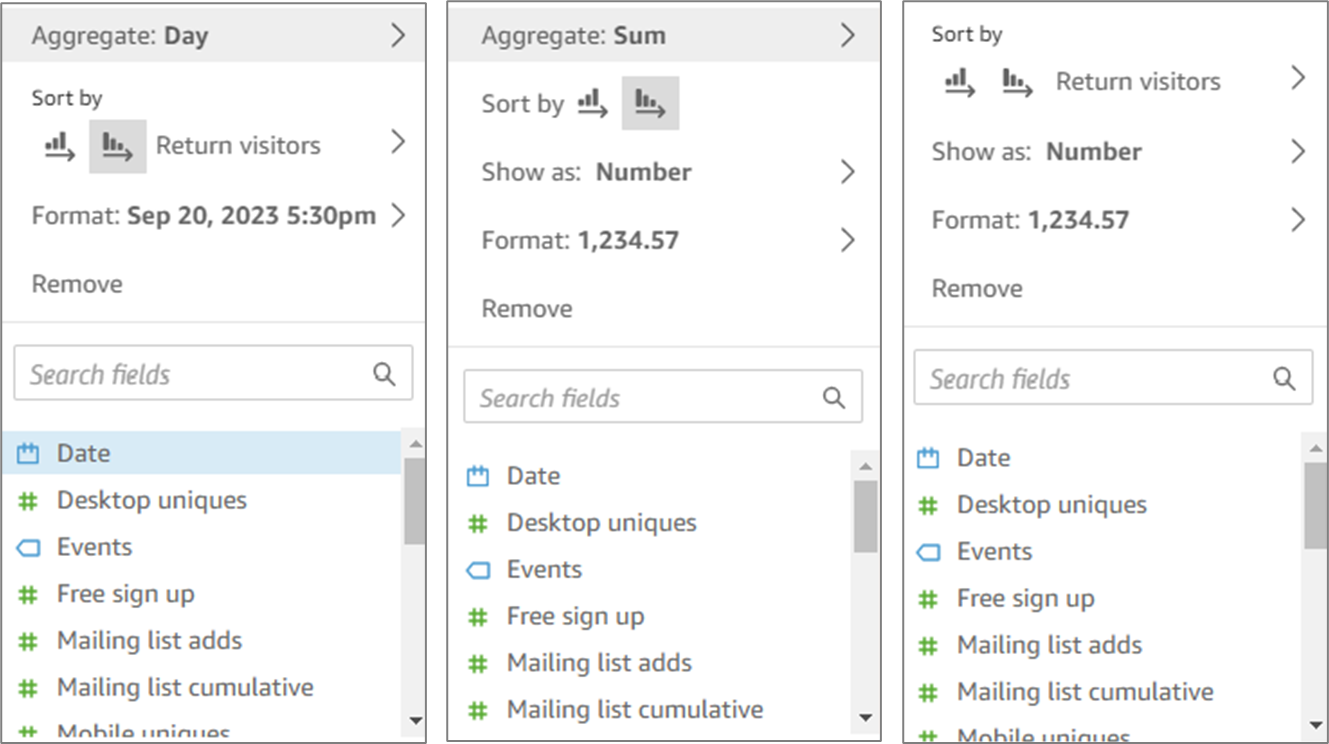Figure 13: Dropdown Options

### Aggregating

(See Figure 14)

You can apply functions to fields to display aggregate information, like the sum of the sales for a given product. You can apply an aggregate function by using the options in either an on-visual editor or a field well (1).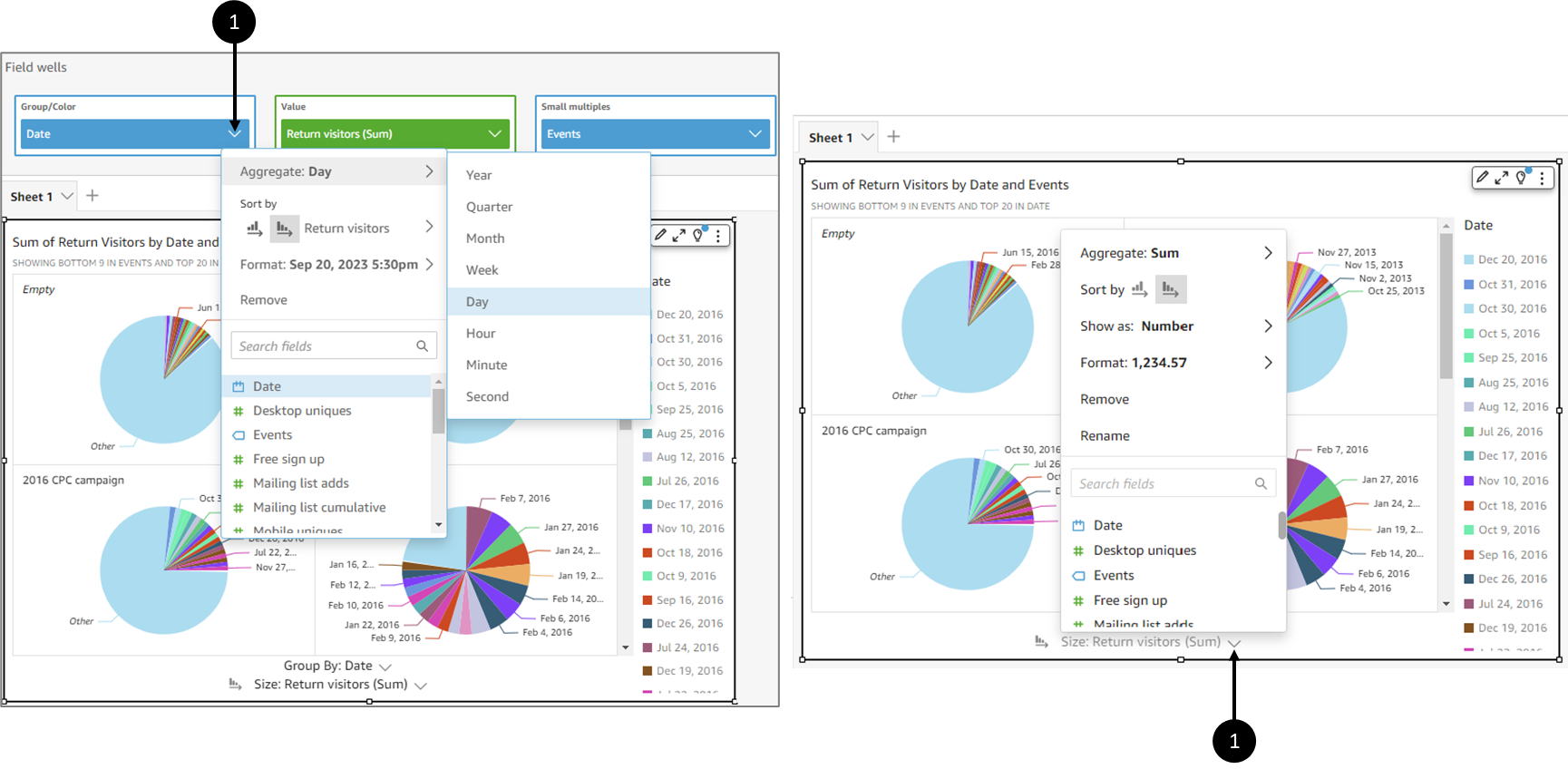Figure 14: Aggregating Options

The following aggregate functions are available in EasySend Analytics:

• Average - calculates the average value for the selected field.
• Count - provides a count of the number of records containing the selected measure for a given dimension. An example is a count of Order IDs by State.
• Distinct Count - provides a count of how many different values are in the selected measure, for the selected dimension or dimensions. An example is a count of Products by Region. A simple count can show how many products are sold for each region. A distinct count can show how many different products are sold for each region. You might have sold 2000 items, but only two different types of items.
• Max - calculates the maximum value for the selected field.
• Min - calculates the minimum value for the selected field.
• Median - calculates the median value of the specified measure, grouped by the chosen dimension or dimensions.
• Sum - totals all the values for the selected field.
• Standard Deviation - calculates the standard deviation of the set of numbers in the specified measure, grouped by the chosen dimension or dimensions, based on a sample or a biased population.
• Variance - calculates the variance of the set of numbers in the specified measure, grouped by the chosen dimension or dimensions, based on a sample or a biased population.
• Percentile - computes the nth percentile of the specified measure, grouped by the chosen dimension or dimensions.

### Sorting

(See Figure 15 and Figure 16)

You can sort data using multiple methods for most visual types. You can choose the sort order of on-visual data by using the quick sort option (1) or Field wells (2).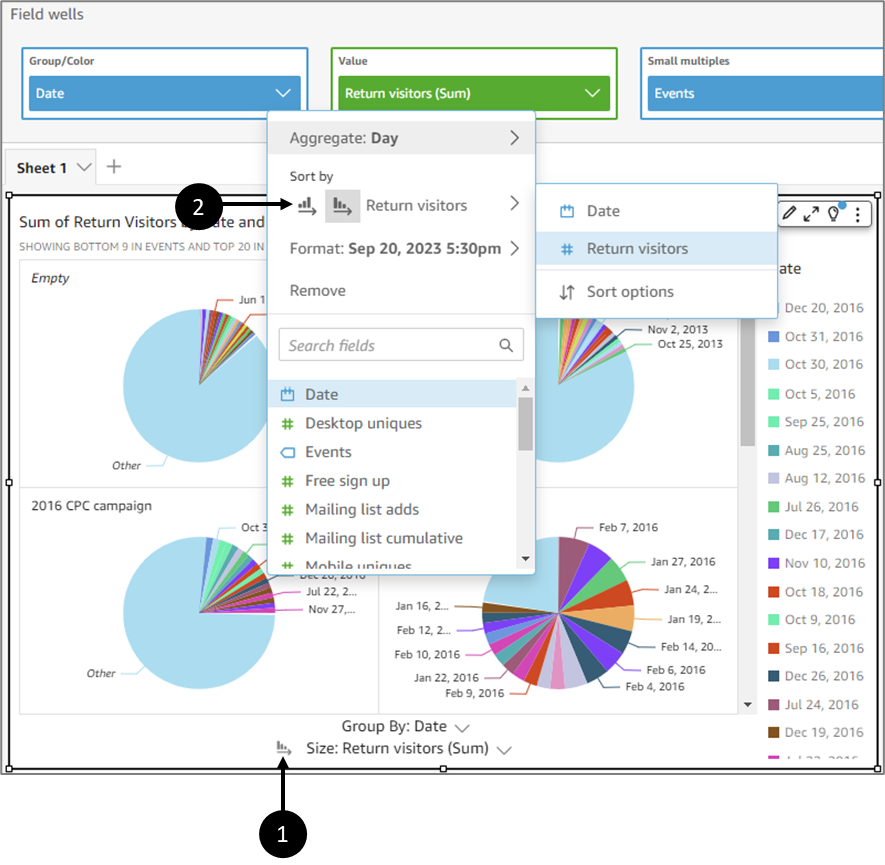Figure 15: Sorting Options

Sorting data on a visual can be done by clicking the label (1) for the field you want to sort, and clicking one of the sort icons (2):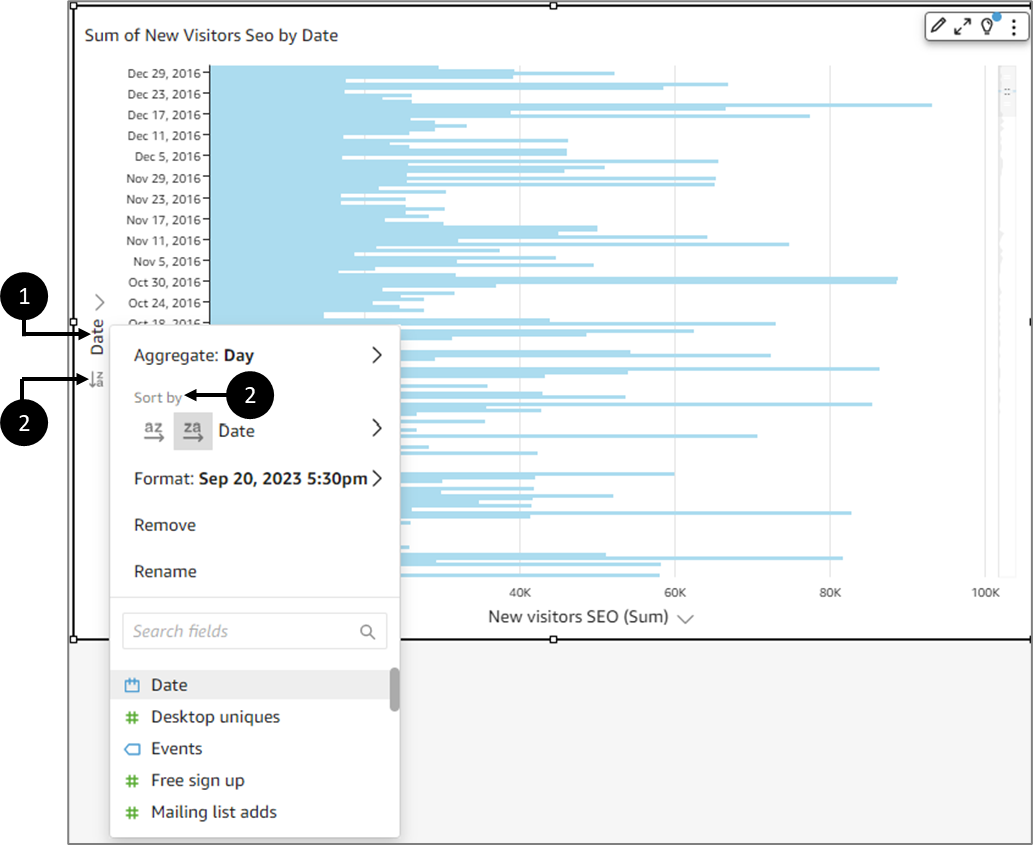Figure 16: Sorting Data on a Visual

### Sorting Data by an Off-Visual Metric

(See Figure 17 and Figure 18)

To use Field wells to sort data by an off-visual metric, perform the following steps:Figure 17: Sorting Data by an Off-Visual Metric

1. Click a visual (1).
2. Expand the Field wells (2).
3. Choose a sorting-supported field and click its dropdown (3).
4. Hover over Sort by (4).
5. Click Sort options (5).

Result:

The Sort options pane appears on the left side of the screen: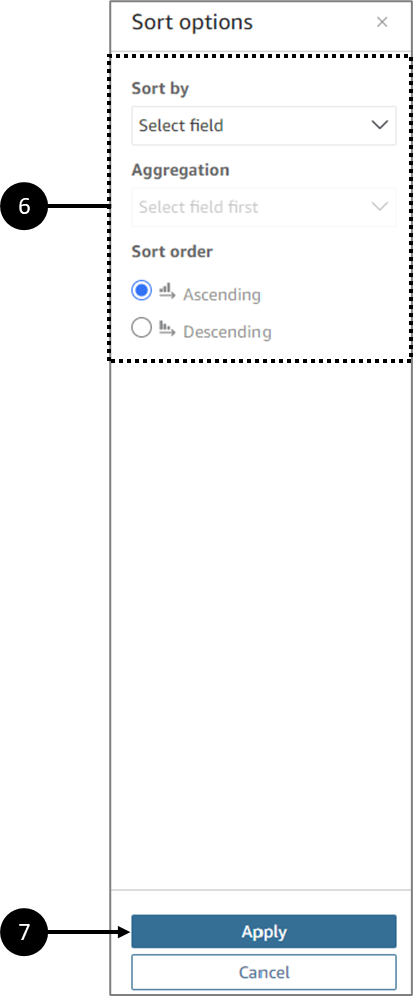Figure 18: Sort options

1. On the Sort options pane, sort by specific fields, choose an Aggregation and a Sort order, Ascending or Descending (6).
2. Click Apply (7) to save the changes.

### Display and Formatting Options

(See Figure 19 and Figure 20)

You can set display and formatting options using the Field wells. Display (Show as) (1) and formatting (Format) (2) options vary based on the field's data type.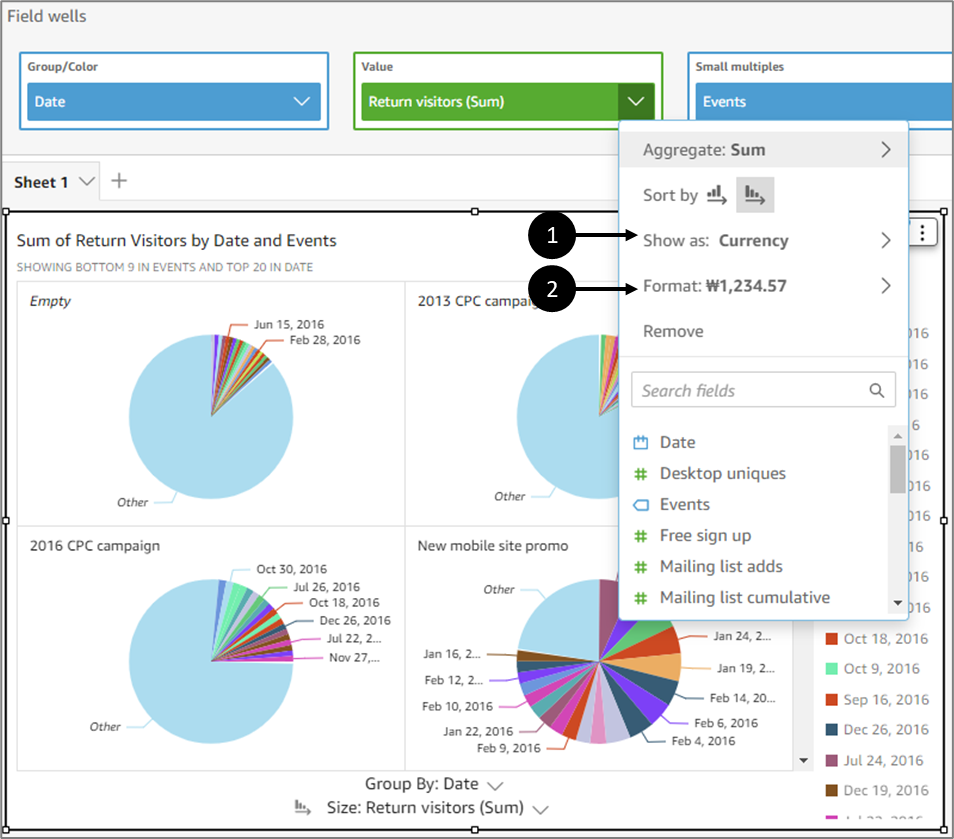Figure 19: Show as and Format

If you do not see an option that you want to use, choose More formatting options from the context menu.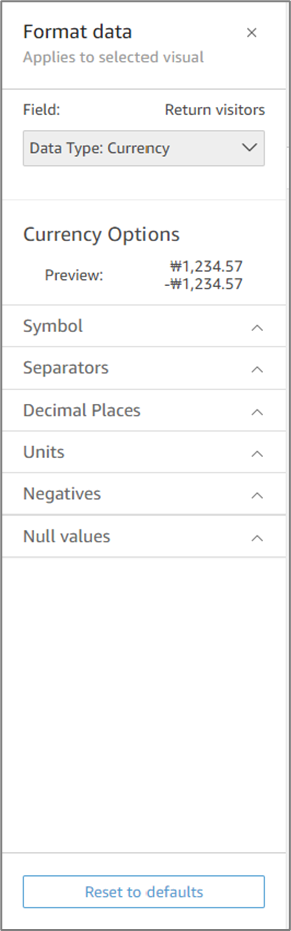Figure 20: Format Data

## Fields List Options

(See Figure 21)

Each field from the Fields list has a few options:

• Add to visual/Remove from visual
• Add a filter for this field
• Show as
• Format
• Count Format
• Convert to measure/dimension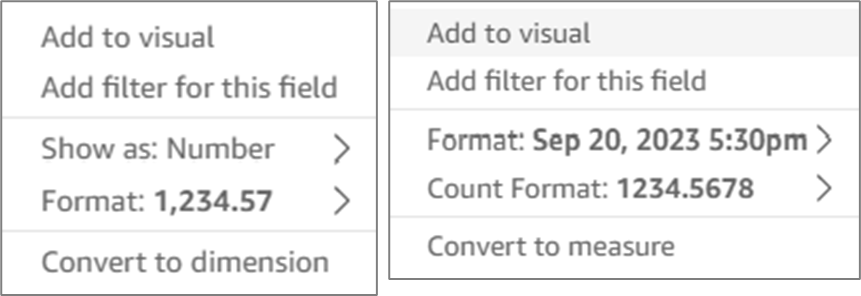Figure 21: Fields List Options

(See Figure 22 to Figure 24)

Datasets that were created using Model data items from your environment can be updated by adding calculated fields.

NOTE
To learn how to create datasets, click here.

You can add calculations based on dataset fields and built-in functions by clicking the  + Add button (1) and the Add calculated field option (2):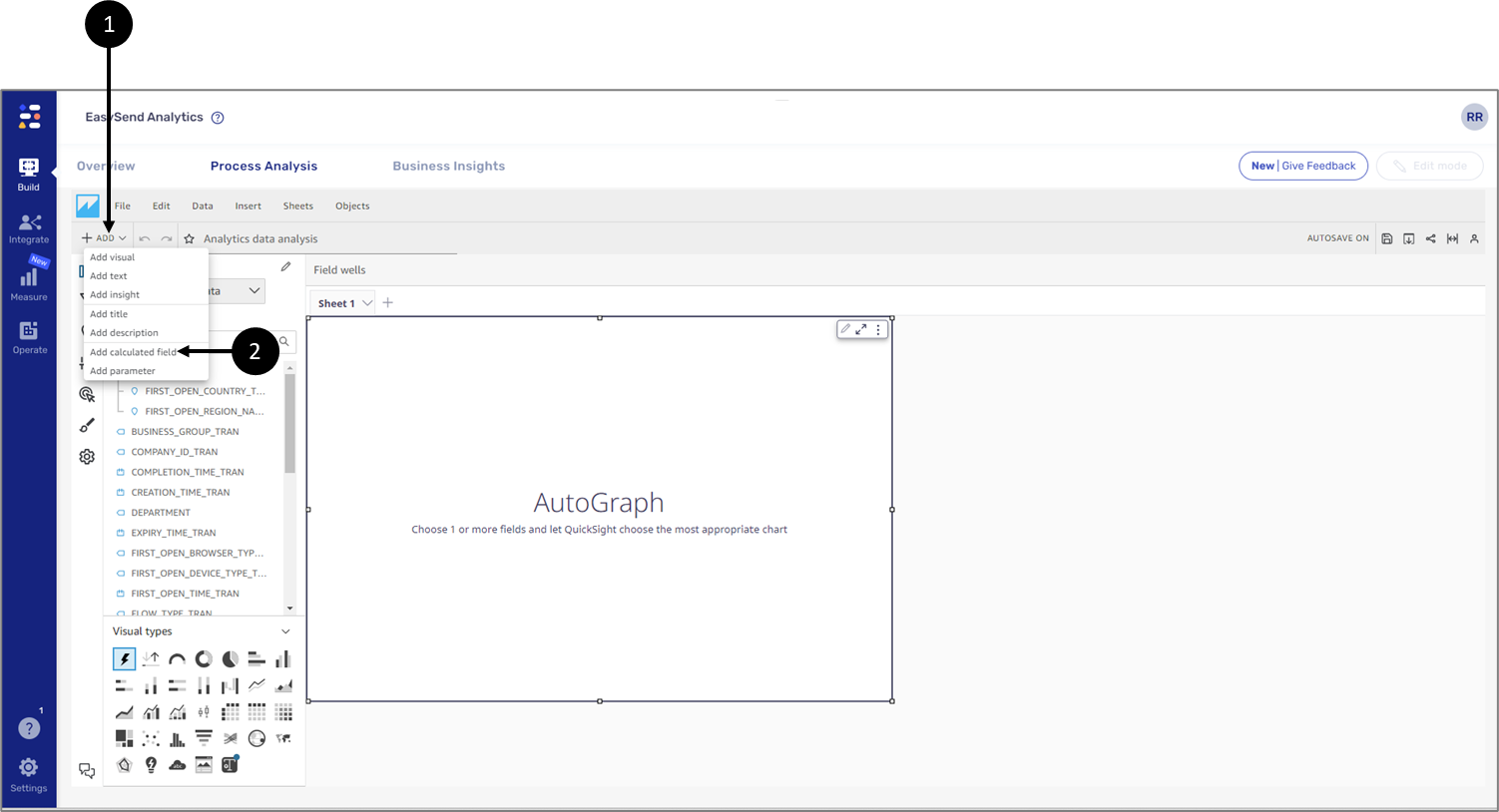Figure 22: Add calculated field Option

After clicking the Add calculated field, an editor screen appears. You can use the different fields, parameters, and built-in functions to create new calculation formulas. For example, you can add a calculation of the average number of children: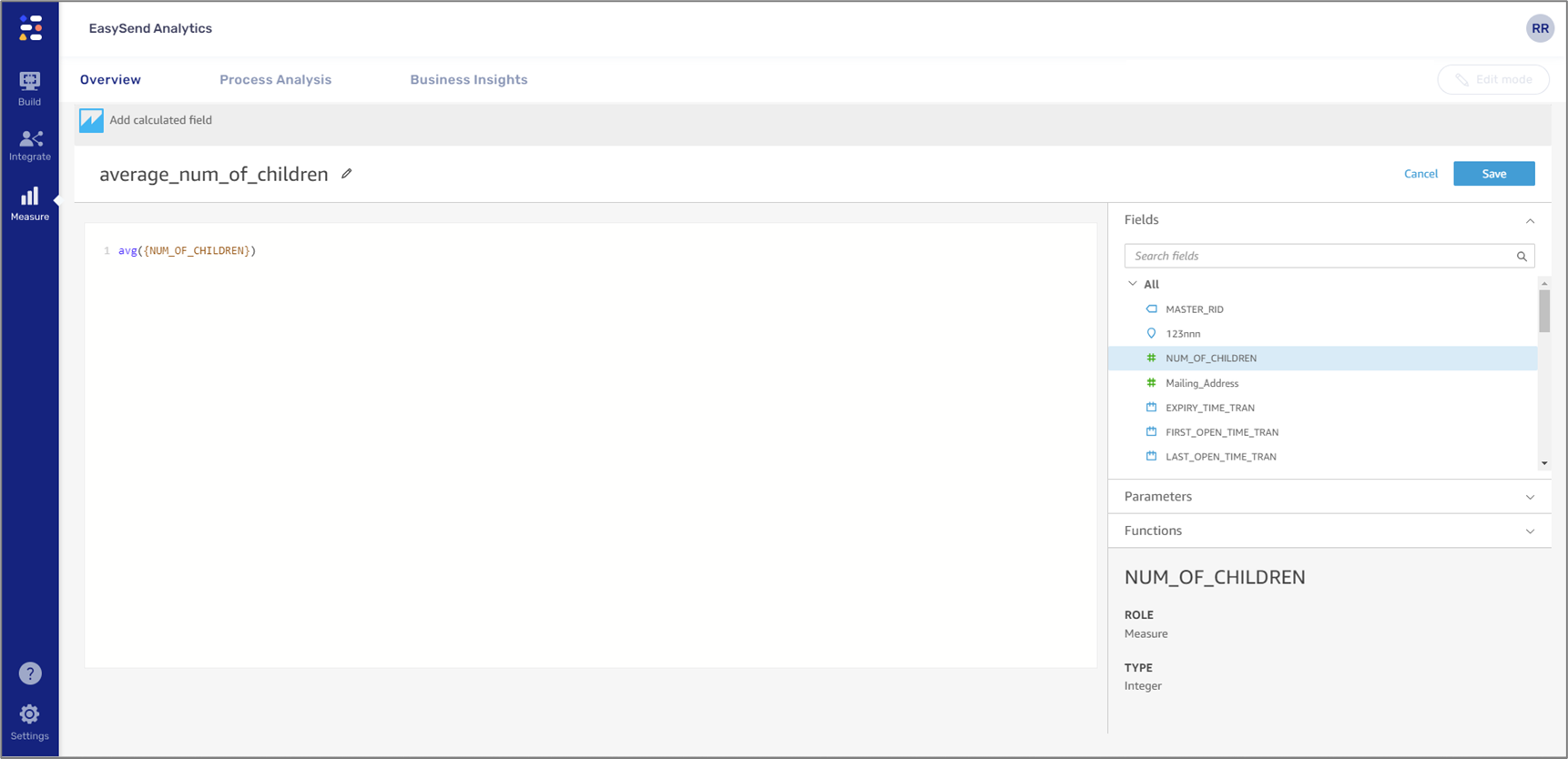Figure 23: Usage Example

Clicking Save will apply the calculation and close the editor. The calculation will appear as a new dataset field (3):

NOTE
You must provide a name before clicking the Save button.